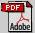Applied Rheology: PublicationsSimple and Accurate Formulas for Flow-Curve Recovery from Couette Rheometer Data

Appl. Rheol. 16:6 (2006) 321-328

Abstract: In Couette rheometry, most of the current flow-curve recovery algorithms require the explicit numerical differentiation of the measured angular velocity data. The exceptions and popular choices, because it avoids the need for a numerical differentiation, are the parallel plate approximation (cf. Bird et al. , Table 10.2-1) and the simplest of the formulas given in Krieger and Elrod . However, their applicability is limited to narrow gap rheometer data. In this paper, equally simple formulas are presented which are exact for Newtonian fluids, do not involve a numerical differentiation and are consistently more accurate than the simple formulas mentioned above. They are based on a generalization of the Euler-Maclaurin sum formula solution of the Couette viscometry equation given in Krieger and Elrod. As well as illustrating the improved accuracy for the recovery of flow-curves for fluids with and without a yield-stress, details about more general and accurate formulas for flow-curve recovery from Couette rheometry data are given. The situation for the recovery of flow-curves from wide gap rheometery measurements is also discussed. © 2006 Applied Rheology.

-- full text PDFavailable for subscribers --

-- open access PDF extractavailable for non-subscribers --